# Subtraction Videos

## Subtracting Mixed Numbers Word Problem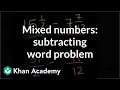Sal of Khan Academy explains how to simplify word problems to the basic numerical question. Get [...]

## Addition and Subtraction with Significant Figures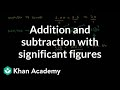Sal from Khan academy explains how to add and subtract with the right level of precision [...]

## Adding and Subtracting Polynomials 3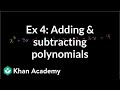Sal from Khan Academy tackles more difficult examples of adding and subtracting polynomials. This more challenging [...]

## Adding and Subtracting Rational Expressions 3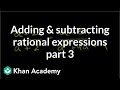Sal from Khan Academy makes adding and subtracting rational expressions easy by explaining how to simplify [...]

## Adding and Subtracting Rational Expressions 2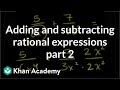Sal of Khan Academy explains how to add and subtract more complicated rational expressions. Get more [...]

## Adding and Subtracting Polynomials 2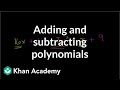Sal from Khan Academy explains how to simplify a complicated polynomial. Learn how to add and [...]

## Subtracting Decimals Word Problem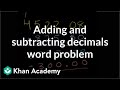Sal from Khan Academy demonstrates how to subtract decimals in a word problem. Apply your knowledge [...]

## Subtracting Decimals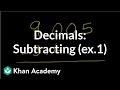Sal of Khan Academy explains how simple subtracting decimals can be. He removes the confusion that [...]

## Adding and Subtracting Rational Expressions 1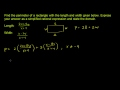Get a great example of how to add and subtract rational expressions through this clear and [...]

## Adding and Subtracting Negative Numbers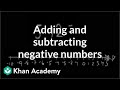Khan Academy makes confusion about adding and subtracting negative numbers a thing of the past. A [...]